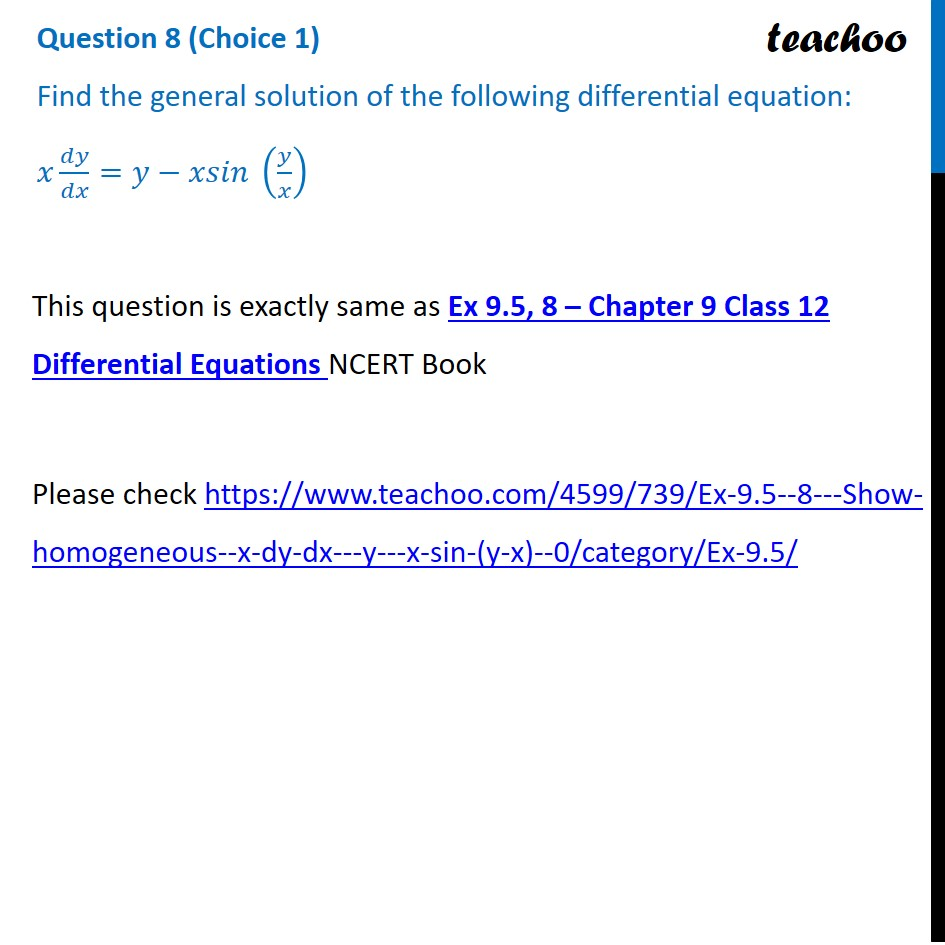CBSE Class 12 Sample Paper for 2022 Boards (For Term 2)

Class 12
Solutions of Sample Papers and Past Year Papers - for Class 12 Boards

## Find the general solution of the following differential equation:x dy/dx =𝑦−𝑥𝑠𝑖𝑛 (𝑦/𝑥)

This question is exactly same Ex 9.5, 8 Chapter 9 Class 12 - Differential Equations

##(adsbygoogle = window.adsbygoogle || []).push({});

### Transcript

Question 8 (Choice 1) Find the general solution of the following differential equation: 𝑥 𝑑𝑦/𝑑𝑥=𝑦−𝑥𝑠𝑖𝑛 (𝑦/𝑥) This question is exactly same as Ex 9.5, 8 – Chapter 9 Class 12 Differential Equations NCERT Book Please check https://www.teachoo.com/4599/739/Ex-9.5--8---Show-homogeneous--x-dy-dx---y---x-sin-(y-x)--0/category/Ex-9.5/